Next: Exercises Up: Vector Algebra and Vector Previous: Grad Operator

Curvilinear Coordinates

In the cylindrical coordinate system, the Cartesian coordinatesand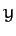are replaced by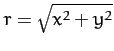and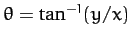. Here,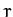is the perpendicular distance from the-axis, andthe angle subtended between the perpendicular radius vector and the-axis--see Figure A.113. A general vector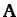is thus written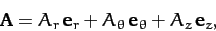(1372)

where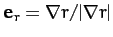and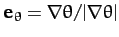--see Figure A.113. Note that the unit vectors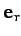,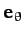, and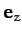are mutually orthogonal. Hence,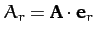, etc. The volume element in this coordinate system is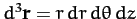. Moreover, the gradient of a general scalar field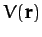takes the form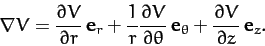(1373)

In the spherical coordinate system, the Cartesian coordinates,, andare replaced by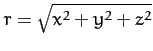,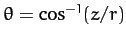, and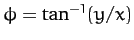. Here,is the radial distance from the origin,the angle subtended between the radius vector and the-axis, and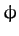the angle subtended between the projection of the radius vector onto the-plane and the-axis--see Figure A.114. Note thatandin the spherical system are not the same as their counterparts in the cylindrical system. A general vectoris written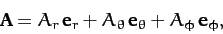(1374)

where,, and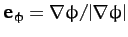. The unit vectors,, and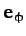are mutually orthogonal. Hence,, etc. The volume element in this coordinate system is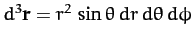. Moreover, the gradient of a general scalar fieldtakes the form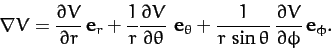(1375)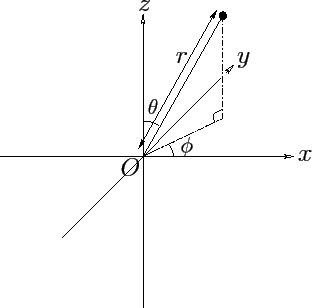Next: Exercises Up: Vector Algebra and Vector Previous: Grad Operator
Richard Fitzpatrick 2011-03-31